PRIMARY

3 - 6

INTERMEDIATE

7 - 8

SECONDARY

9 - 11

Home

# Quotient Rule

Theory & Practice Questions.

## Lesson

#### [Formula]

If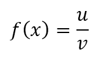where u and v are both functions of x, then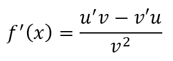## Example

Differentiate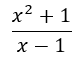## Solution

Recall that ifwhere u and v are both functions of x, thenu = x² + 1      v = x-1

u' = 2x           v' = 1

Using this, we get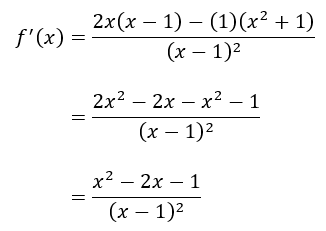## Question

Differentiate the function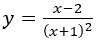## Explanation

Use the quotient rule of differentiation, which says that ifwhere u and v are both functions of x, thenu = x - 2      v = (x+1)²

u' = 1           v' = 2(x+1)

Using the formula, we have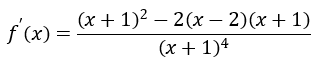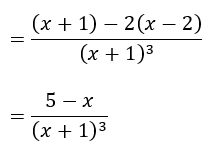School Year